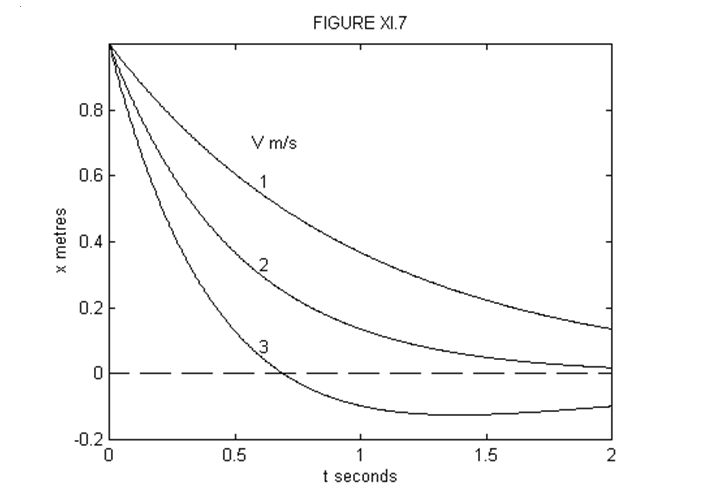$$\require{cancel}$$

# 11.5ii: Heavy damping- $$\gamma > 2\omega_{0}$$

•• Contributed by Jeremy Tatum
• Emeritus Professor (Physics & Astronomy) at University of Victoria

The motion is given by Equations 11.5.4 and 11.5.6 where, this time, $$k_{1}$$ and $$k_{2}$$ are each real and negative. For convenience, I am going to write $$\lambda_{1}=-k_{1}$$ and $$\lambda_{2}=-k_{2}$$. $$\lambda_{1}$$ are $$\lambda_{2}$$ both real and positive, with $$\lambda_{2}$$ > $$\lambda_{1}$$ given by

$\lambda_{1}=\frac{1}{2}\gamma-\sqrt{(\frac{1}{2}\gamma)^{2}-\omega_{0}^{2}},\quad \lambda_{2}=\frac{1}{2}\gamma+\sqrt{(\frac{1}{2}\gamma)^{2}-\omega_{0}^{2}} \label{11.5.19}\tag{11.5.19}$

The general solution for the displacement as a function of time is

$x=Ae^{-\lambda_{1}t}+Be^{-\lambda_{2}t}. \label{11.5.20}\tag{11.5.20}$

The speed is given by

$\dot{x}=-A\lambda_{1}e^{-\lambda_{1}t}-B\lambda_{2}e^{-\lambda_{2}t}. \label{11.5.21}\tag{11.5.21}$

The constants $$A$$ and $$B$$ depend on the initial conditions. Thus:

$x_{0}=A+B \label{11.5.22}\tag{11.5.22}$

and

$(\dot{x})_{0}=-(A\lambda_{1}+B\lambda_{2}). \label{11.5.23}\tag{11.5.23}$

From these, we obtain

$A=\frac{(\dot{x})_{0}+\lambda_{2}x_{0}}{\lambda_{2}-\lambda_{1}},\qquad B=-[\frac{(\dot{x})_{0}+\lambda_{1}x_{0}}{\lambda_{2}-\lambda_{1}}]. \label{11.5.24}\tag{11.5.24}$

Example $$\PageIndex{1}$$

$$x_{0}\neq0,\quad(\dot{x})_{0}=0.$$

$x=\frac{x_{0}}{\lambda_{2}-\lambda_{1}}(\lambda_{2}e^{-\lambda_{1}t}-\lambda_{1}e^{-\lambda_{2}t}). \label{11.5.25}\tag{11.5.25}$

Figure XI.4 shows $$x\quad:\quad t$$ for $$x_{0}$$ = 1 m, $$\lambda_{1}$$ = 1 s-1, $$\lambda_{2}$$ = 2 s-1.

The displacement will fall to half of its initial value at a time given by putting $$\frac{x}{x_{0}}=\frac{1}{2}$$ in Equation $$\ref{11.5.25}$$. This will in general require a numerical solution. In our example, however, the equation reduces to $$\frac{1}{2}=2e^{-t}-e^{-2t}$$ and if we let $$u=e^{-t}$$, this becomes $$u^{2}-2u+\frac{1}{2}=0$$. The two solutions of this are $$u=1.707107$$ or $$0.292893$$. The first of these gives a negative t, so we want the second solution, which corresponds to $$t= 1.228$$ seconds.

The velocity as a function of time is given by

$\dot{x}=-\frac{\lambda_{1}\lambda_{2}x_{0}}{\lambda_{2}-\lambda_{1}}(e^{-\lambda_{1}t}-e^{\lambda_{2}t}). \label{11.5.26a}\tag{11.5.26a}$

This is always negative. In figure XI.5, is shown the speed, which is $$|\dot{x}|$$ as a function of time, for our numerical example. Those who enjoy differentiating can show that the maximum speed is reached in a time $$-\dot{x}$$ and that the maximum speed is $$\frac{\lambda_{1}\lambda_{2}x_{0}}{\lambda_{2}-\lambda_{1}}[(\frac{\lambda_{1}}{\lambda_{2}})^{\frac{\lambda_{2}}{\lambda_{2}-\lambda_{1}}}-(\frac{\lambda_{1}}{\lambda_{2}})^{\frac{\lambda_{1}}{\lambda_{2}-\lambda_{1}}}]$$. (Are these dimensionally correct?) In our example, the maximum speed, reached at $$t=\ln 2=0.6931$$ seconds, is 0.5 m s-1.Example $$\PageIndex{2}$$

$$x_{0}=0,\quad (\dot{x})_{0}=V(>0)$$.

In this case it is easy to show that

$x=\frac{V}{\lambda_{2}-\lambda_{1}}(e^{-\lambda_{1}t}-e^{-\lambda_{2}t}). \label{11.5.26b}\tag{11.5.26b}$

It is left as an exercise to show that $$x$$ reaches a maximum value of $$\frac{V}{\lambda_{2}}(\frac{\lambda_{1}}{\lambda_{2}})^{\frac{\lambda_{1}}{\lambda_{2}-\lambda_{1}}}$$ when $$t=\frac{\ln(\frac{\lambda_{2}}{\lambda_{1}})}{\lambda_{2}-\lambda_{1}}$$. Figure XI.6 illustrates Equation $$\ref{11.5.26a}$$ for $$\lambda_{1}$$ = 1 s-1, $$\lambda_{2}$$ = 2 s-1, $$V$$ = 5 m s-1. The maximum displacement of 1.25 m is reached when $$t = \ln 2 = 0.6831$$ s. It is also left as an exercise to show that equation $$\ref{11.5.26a}$$ can be written

$x=\frac{2Ve^{-\frac{1}{2}\lambda t}}{\lambda_{2}-\lambda_{1}}\sinh (\frac{1}{4}\gamma^{2}-\omega_{0}^{2}). \label{11.5.27}\tag{11.5.27}$Example $$\PageIndex{3}$$

$$x_{0}\neq 0,\quad(\dot{x})_{0}=-V$$.

This is the really exciting example, because the suspense-filled question is whether the particle will shoot past the origin at some finite time and then fall back to the origin; or whether it will merely tamely fall down asymptotically to the origin without ever crossing it. The tension will be almost unbearable as we find out. In fact, I cannot wait; I am going to plot $$x$$ versus $$t$$ in figure XI.7 for $$\lambda_{1}$$ = 1 s-1, $$\lambda_{2}$$ = 2 s -1, $$x_{0}$$ = 1 m, and three different values of $$V$$, namely 1, 2 and 3 m s-1.We see that if $$V$$ = 3 m s-1 the particle overshoots the origin after about 0.7 seconds. If $$V$$ = 1 m s-1, it does not look as though it will ever reach the origin. And if $$V$$ = 2 m s-1, I'm not sure. Let's see what we can do. We can find out when it crosses the origin by putting $$x$$= 0 in Equation $$\ref{11.5.20}$$, where $$A$$ and $$B$$ are found from Equations $$\ref{11.5.24}$$ with $$(\dot{x})_{0}=-V$$. This gives, for the time when it crosses the origin,

$t=\frac{1}{\lambda_{2}-\lambda_{1}}\ln(\frac{V-\lambda_{1}x_{0}}{V-\lambda_{2}x_{0}}). \label{11.5.28}\tag{11.5.28}$

Since $$\lambda_{2} > \lambda_{1}$$, this implies that the particle will overshoot the origin if $$V > \lambda_{2}x_{0}$$, and this in turn implies that, for a given $$V$$, it will overshoot only if

$\gamma < \frac{\frac{V^{2}}{x_{0}^{2}}+\omega_{0}^{2}}{\frac{V}{x_{0}}}. \label{11.5.29}\tag{11.5.29}$

For our example, $$\lambda_{2}x_{0}$$= 2 m s-1, so that it just fails to overshoot the origin if $$V$$= 2 m s-1. For $$V$$ = 3 m s-1, it crosses the origin at $$t=\ln 2=0.6931$$ s. In order to find out how far past the origin it goes, and when, we can do this just as in

I make it that it reaches its maximum negative displacement of -0.125 m at $$t = \ln 4 = 1.386$$ s.